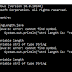In the last tutorial, we have learned how to check a stringor not. In this tutorial, we will learn how to find the string length in java. We can find string length in java using the length() method of the String class. This method returns the length of the string. Remember that the length variable is used in the array whereas the length() method is used in the string. If you try to use the length variable to find the string length then complier will get a compile-time error.

Syntax: The syntax of the string class length() method is given below:
``````
public int length()``````

## Example of Java String length() Method:

``````
class StringLength{
public static void main(String args[]){
String str1 = "Javastudypoint";
String str2 = "Prashant";
//14 is the length of the str1.
System.out.println("The length of the str1 is: "+str1.length());
//8 is the length of the str2.
System.out.println("The length of the str2 is: "+str2.length());
}
}
``````

Output:
``````
The length of the str1 is: 14
The length of the str2 is: 8
``````

Let's see an example of what happened if we are using length variable instead of length() method in case of a string.  If you do so, then the compiler will get a compile-time error saying cannot find the symbol. Let's see the example.
``````
class StringLength{
public static void main(String args[]){
String str1 = "Javastudypoint";
String str2 = "Prashant";
//using length variable instead of length().
System.out.println("The length of the str1 is: "+str1.length);
//using length variable instead of length().
System.out.println("The length of the str2 is: "+str2.length);
}
}
``````
How to find String length in Java using length method?Reviewed by Prashant Srivastava on January 04, 2020 Rating: 5